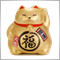# Generating new strategies automatically200

Is there a way to generate new strategies automatically using any methods in artificial intelligent? Any such articles would be greatly helpful.

Reasons are that there are exponentially many indicators we can combine, but it is hard to get one with lots of trades while having good trading results.181

I think besides of an algorithm, you have to learn python too.2887

well, if one would believe on math & science, forex market could be considered as "predictable" using probability ;)

Some fun to explore here ...
https://seeing-theory.brown.edu/basic-probability/index.html

According to Wikipedia
https://en.wikipedia.org/wiki/Probability

Probability is the branch of mathematics concerning numerical descriptions of how likely an event is to occur or how likely it is that a proposition is true. Probability is a number between 0 and 1, where, roughly speaking, 0 indicates impossibility and 1 indicates certainty.

Looking forward for constructive ideas and thoughts....

enjoy.Basic Probability
• seeing-theory.brown.edu
Randomness is all around us. Probability theory is the mathematical framework that allows us to analyze chance events in a logically sound manner. The probability of an event is a number indicating how likely that event will occur. This number is always between 0 and 1, where 0 indicates impossibility and 1 indicates certainty. A classic...